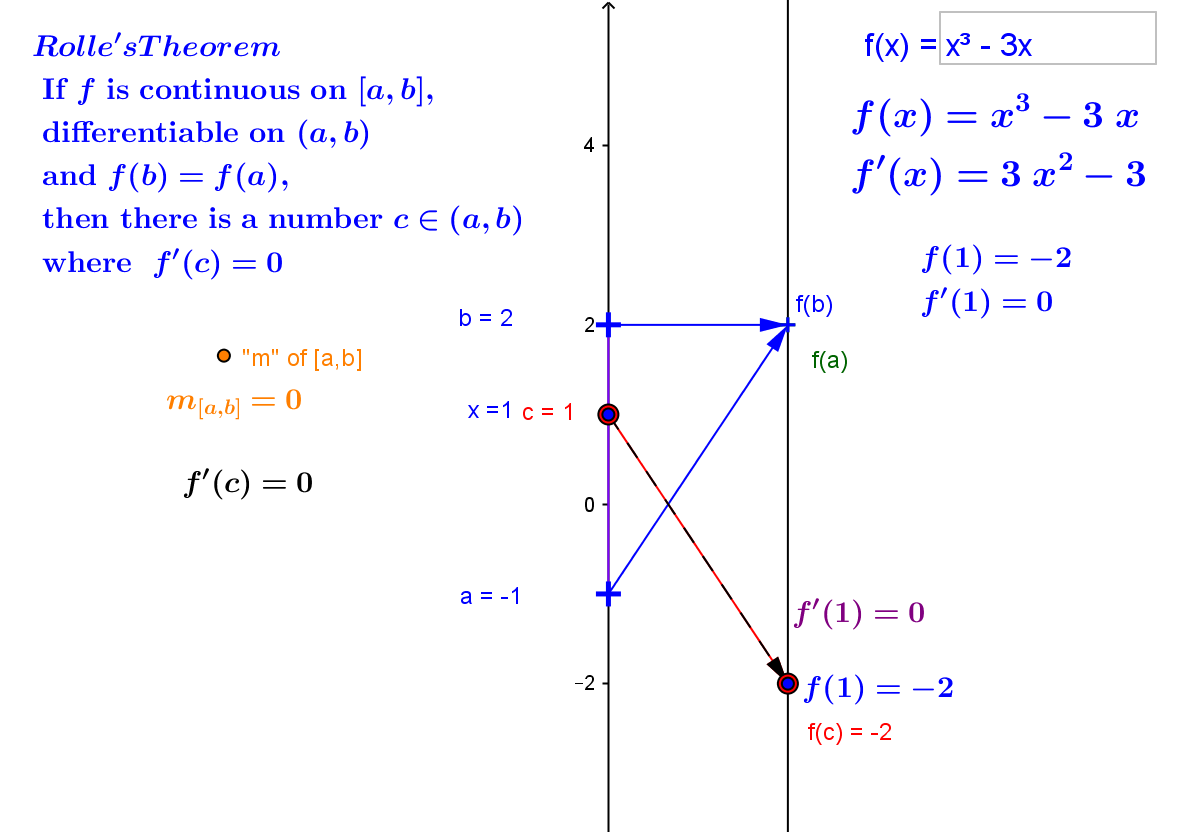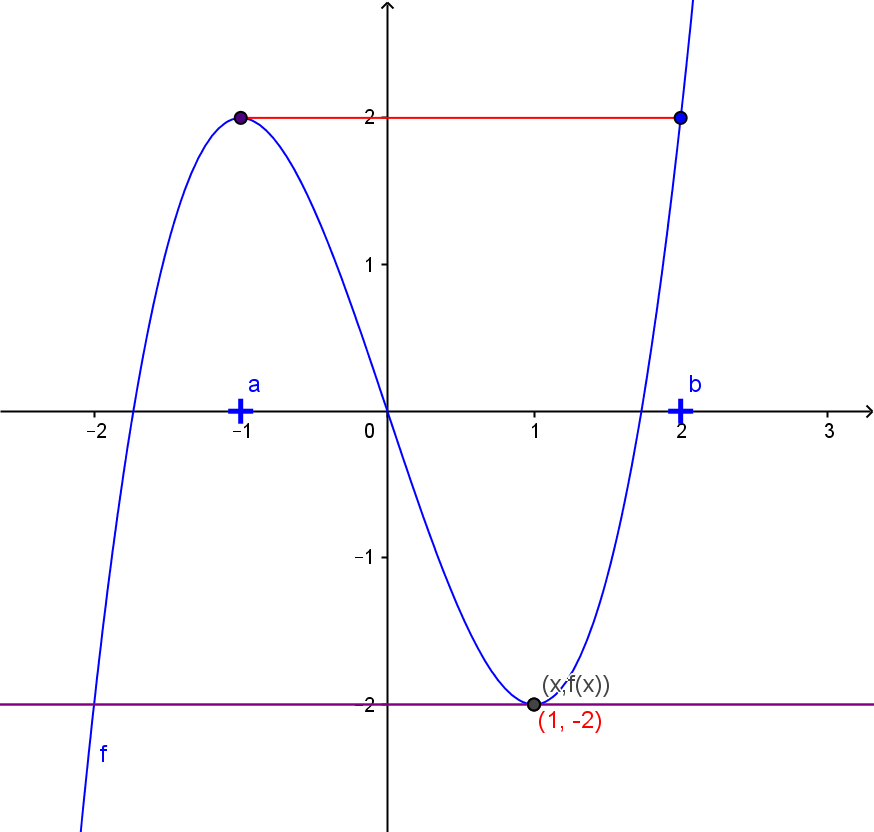Theorem: CCD.MVT. The Mean Value Theorem.
If $f$ is a continuous function on the interval $[a,b]$ and a differentiable function on the interval $(a,b)$, then there is a number $c \in (a,b)$  where  $$f'(c) = \frac{f(b) - f(a)}{b-a}$$ or $$f(b) = f(a) + f'(c) (b-a)$$ .
Theorem: CCD.RT: Rolle's Theorem.
If $f$ is a continuous function on the interval $[a,b]$ and a differentiable function on the interval $(a,b)$ and $f(b)=f(a)$, then there is a number $c \in (a,b)$  where  $f'(c) = 0$

Proof of the Mean Value Theorem:
The idea of this proof can be thought of as comparing the function $f$ with the linear function that passes through $(a,f(a))$ and $(b,f(b))$ or that has focus point determined by the arrows <$a,f(a)$> and <$b,f(b)$> and has slope or magnification factor $\frac{f(b) - f(a)}{b-a}$. For the remainder of the argument we will denote the number by $M$. We consider the difference of these two functions and notice from the figures which we use to visualize the situation that this difference seems to be greatest precisely at the point where the tangent line is parallel to the secant line and the derivative vector is equal to the magnification vector..
To discuss the difference we let $g(t) = f(a) + M \cdot (t - a) - f(t)$ for all $t$ in $[a,b]$.

Since $f$ and the linear function are continuous on $[a,b]$ and differentiable on $(a,b)$, $g$ is continuous on $[a,b]$ and differentiable on $(a,b)$ as well. By the continuity of $g$ we can apply the extreme value theorem to say that for some $c_*$ and $c^*$ in $[a,b]$ we have extreme values for $g$ on the interval. If either of these points is inside the interval then we can apply the critical point theorem to $g$ to say that $g'(c_*) = 0$ or $g'(c^*)= 0$.

But when we compute we find $g'(t) = M - f'(t)$.  Thus we have either $M - f'(c_*) = 0$, in which case $f'(c_*)= 0$, or $M - f'(c^*) = 0$,  in which case $f'(c^*) = M$. This means that if either $c_*$ or $c^*$ are in the open interval $(a,b)$ the theorem's conclusion is satisfied.
This leaves only the case when the endpoints, $a$ and $b$ are the points where the extremes of $g$ occur.  Now we evaluate $g$ at these two points.
$g(a) = f(a) + M\cdot (a - a) - f(a) = 0$    while $g(b) = f(a) + M\cdot(b - a) - f(b) = f(a) + \frac {f(b)-f(a)}{b-a} \cdot (b - a) - f(a) = 0$.
But if both the maximum and minimum values of $g$ are 0, then $g(t) = 0$ for all $t$ in $[a,b]$.
Looking back at the definition of $g$, we see that in this case the last equation means that $f(t)=f(a) + M \cdot (t - a)$ for all $t$ in $[a,b]$.
Thus we conclude that in this case $f'(c) = M$ for not just some, but for any $c$ in $[a,b]$.

In summary , we see that in any case the conclusion of the Mean Value Theorem is satisfied at some point $c$ in $(a,b)$.      EOP
Here is a GeoGebra applet that visualizes some aspects of the proof . It also provides with a dynamic tool that allows you to investigate lines parallel to the secant line to see how these lines can be moved up or down to find the number $c$ that exists according to the theorem where $f '(c) = \frac{f(b) - f(a)}{b-a}$.

Rolle's Theorem is sometimes proven before the Mean Value Theorem even though as you can see it is merely a special case of the MVT.
Proof of Rolle's Theorem assuming the MVT:  In Rolle's theorem the assumptions of the MVT are all assumed along with the added assumption that $f(b) = f(a)$. This last assumption implies that $f(b) - f(a) = 0$ , so by applying the MVT to $f$ gives the claimed result.
Interpretations: 1. Motion. Suppose $f(t)$ is the position of an object moving continuously over a time interval $[a,b]$ with a velocity at every moment between time $a$ and $b$. The assumptions for Rolle's Theorem also assume that $f(a) = f(b)$, i.e., from the point of view of motion at times $a$ and $b$, there seems to be no change in position. Rolle's Theorem can be interpreted in this situation as meaning that at some moment in time $c$ between time $a$ and $b$ the instantaneous velocity of the object will be equal to $0$, i.e. , at the instant $c$ the object appeared to be stopped.
2. Graphical. Consider the graphof the function $f$ over the interval $[a,b]$. Here the assumption that $f(a) = f(b)$ means that the secant line between $(a,f(a))$ and $(b,f(b))$ is horizontal with its slope $0$. Rolle's Theorem can be interpreted in this situation as meaning that for some $c$ between $a$ and $b$, the slope of the tangent line at that point will be $0$. To express it geometrically, the tangent line will be horizontal at some point on the graph of $f$ . .
3. Economic. If the profit at two levels of production, $a$ and $b$,are equal, then at some level of production between those $a$ and $b$, the marginal profit is $0$.